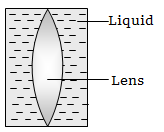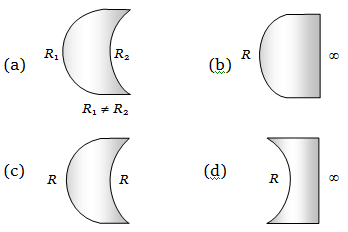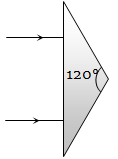The diameter of a plano-convex lens is 6 cm and thickness at the centre is 3 mm. If the speed of light in the material of the lens is $2×{10}^{8}m}{sec}$, the focal length of the lens is

(a) 15 cm                         (b) 20 cm

(c) 30 cm                         (d) 10 cm

Concept Questions :-

Lenses
High Yielding Test Series + Question Bank - NEET 2020

Difficulty Level:

A luminous object is placed at a distance of 30 cm from the convex lens of focal length 20 cm. On the other side of the lens, at what distance from the lens, a convex mirror of radius of curvature 10 cm be placed in order to have an upright image of the object coincident with it?

(a) 12 cm                              (b) 30 cm

(c) 50 cm                              (d) 60 cm

Concept Questions :-

Lenses
High Yielding Test Series + Question Bank - NEET 2020

Difficulty Level:

Shown in the figure here is a convergent lens placed inside a cell filled with a liquid. The lens has focal length + 20 cm when in air and its material has refractive index 1.50. If the liquid has refractive index 1.60, the focal length of the system is(a) + 80 cm                         (b) – 80 cm

(c) – 24 cm                          (d) –160 cm

Concept Questions :-

Lensmakers' formula
High Yielding Test Series + Question Bank - NEET 2020

Difficulty Level:

Which one of the following spherical lenses does not exhibit dispersion? The radii of curvature of the surfaces of the lenses are as given in the diagramsConcept Questions :-

Lenses
High Yielding Test Series + Question Bank - NEET 2020

Difficulty Level:

The size of the image of an object, which is at infinity, as formed by a convex lens of focal length 30 cm is 2 cm. If a concave lens of focal length 20 cm is placed between the convex lens and the image is at a distance of 26 cm from the convex lens, calculate the new size of the image.

(a) 1.25 cm                              (b) 2.5 cm

(c) 1.05 cm                              (d) 2 cm

Concept Questions :-

Lenses
High Yielding Test Series + Question Bank - NEET 2020

Difficulty Level:

An achromatic prism is made by crown glass prism $\left({A}_{c}=19°\right)$ and flint glass prism $\left({A}_{F}=6°\right)$. If ${}_{C}\mu _{v}=1.5$ and ${}_{F}\mu _{v}=1.66$, then resultant deviation for red coloured ray will be

(a) 1.04°                     (b) 5°

(c) 0.96°                      (d) 13.5°

Concept Questions :-

Prisms
High Yielding Test Series + Question Bank - NEET 2020

Difficulty Level:

An isosceles prism of angle 120° has a refractive index of 1.44. Two parallel monochromatic rays enter the prism parallel to each other in air as shown. The rays emerging from the opposite faces :(a) Are parallel to each other

(b) Are diverging

(c) Make an angle $2{\mathrm{sin}}^{-1}\left(0.72\right)$ with each other

(d) Make an angle $2\left\{{\mathrm{sin}}^{-1}\left(0.72\right)-30°\right\}$ with each other

Concept Questions :-

Prisms
High Yielding Test Series + Question Bank - NEET 2020

Difficulty Level:

A ray of light is incident on the hypotenuse of a right-angled prism after travelling parallel to the base inside the prism. If $\mu$ is the refractive index of the material of the prism, the maximum value of the base angle for which light is totally reflected from the hypotenuse is

(a) ${\mathrm{sin}}^{-1}\left(\frac{1}{\mu }\right)$                             (b) ${\mathrm{tan}}^{-1}\left(\frac{1}{\mu }\right)$

(c) ${\mathrm{sin}}^{-1}\left(\frac{\mu -1}{\mu }\right)$                         (d) ${\mathrm{cos}}^{-1}\left(\frac{1}{\mu }\right)$

Concept Questions :-

Total internal reflection
High Yielding Test Series + Question Bank - NEET 2020

Difficulty Level:

A plano-convex lens when silvered in the plane side behaves like a concave mirror of focal length 30 cm. However, when silvered on the convex side it behaves like a concave mirror of focal length 10 cm. Then the refractive index of its material will be

(a) 3.0                                (b) 2.0

(c) 2.5                                (d) 1.5

Concept Questions :-

Lenses
High Yielding Test Series + Question Bank - NEET 2020

Difficulty Level:

A ray of light travels from an optically denser to rarer medium. The critical angle for the two media is C. The maximum possible deviation of the ray will be

(a) $\left(\frac{\mathrm{\pi }}{2}-C\right)$                          (b) 2C

(c) $\mathrm{\pi }-2\mathrm{C}$                             (d) $\mathrm{\pi }-\mathrm{C}$

Concept Questions :-

Total internal reflection